## Deploying containers

This Chromium Project is being considered since it has an Optimized OS to build containers which could be imported as image in a Compute Engine. With this scheme then the engine is able to be assigned as a runner to build inside container.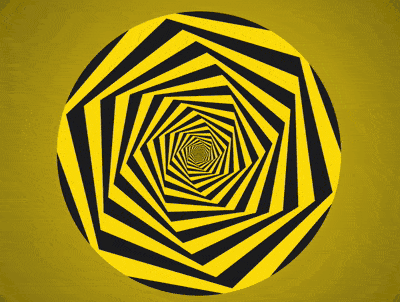The main reason of this requirement is because we will need to build a system that contained as three (3) layers of primes level where the system will always be forced in to the 15+25=40th prime each time we made an access to the next layer.

``````1st layer:
It has a total of 1000 numbers
Total primes = π(1000) = 168 primes

2nd layer:
It will start by π(168)+1 as the 40th prime
It has 100x100 numbers or π(π(10000)) = 201 primes
Total cum primes = 168 + (201-40) = 168+161 = 329 primes

3rd layer:
Behave the same as 2nd layer which has a total of 329 primes
The primes will start by π(π(π(1000th prime)))+1 as the 40th prime
This 1000 primes will become 1000 numbers by 1st layer of the next level
Total of all primes = 329 + (329-40) = 329+289 = 618 = 619-1 = 619 primes - Δ1
``````

Althouht the optimized os is ready with built in images however we will need to place a custom modification since the objective of this OS Project is mainly about optimizing the containers while our project is mainly about the primes behaviour.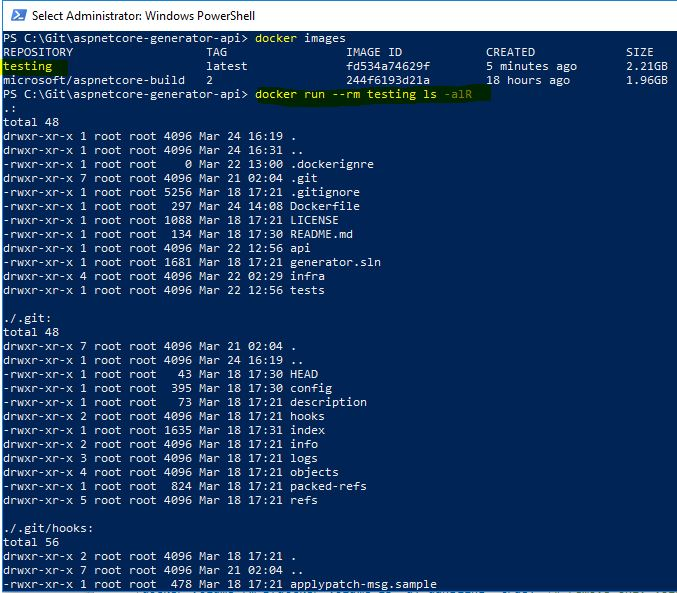By this modification we are going to build the three (3) layers of 19 cells with a cumulative sum of 1, 7 and 19 in sequence. So follow to the scheme then it would get 50 nodes out of the total nodes of 66.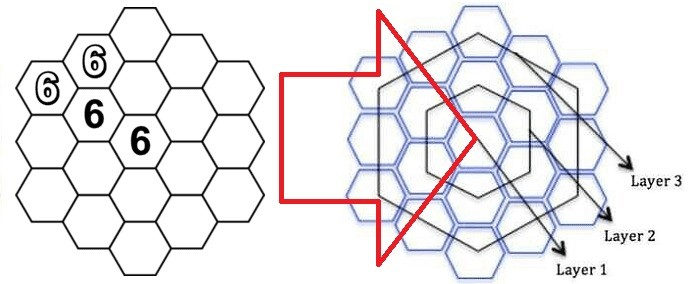Consider that the 1st cell is standing as (Δ1) which is eqivalent to 19. Then as per The Δ(19 vs 18) Scenario the 7 cells would behave as (Δ1 to Δ7) that equivalent with 19 to 25. So by the 3rd and 4th axis it will get 102+66 = 168 primes of π(1000).

``````                                |                              ----------- 5 -----------
|                             |                         |
↓                             ↑                         ↓
|   mapping    |     feeding     |  lexering    |  parsering   |   syntaxing   |  grammaring  |
|------------- 36' --------------|----------------------------36' ----------------------------|
|     19'      |        17'      |      13'     |      11'     |       7'      |       5'     |
+----+----+----+---+----+----+---+---+----+-----+----+----+----+----+----+-----+----+----+----+
|  1 |  2 |  3 | 4 |  5 |  6 | 7 | 8 |  9 |  10 | 11 | 12 | 13 | 14 | 15 |  16 | 17 | 18 | 19 |
+----+----+----+---+----+----+---+---+----+-----+----+----+----+----+----+-----+----+----+----+
|  2 | 60 | 40 | 1 | 30 | 30 | 5 | 1 | 30 | 200 |  8 | 40 | 50 |  1 | 30 | 200 |  8 | 10 | 40 |
+----+----+----+---+----+----+---+---+----+-----+----+----+----+----+----+-----+----+----+----+
↓                             ↑                         |    |
|                             |                         |    |
------------ 10 -------------                          |    |
↓    ↓ |
+----+----+----+---+----+----+---+
|  2 | 60 | 40 | 1 | 30 | 30 | 5 |
+----+----+----+---+----+----+---+
|    | |
2+100 ◄-
-----------------------+----+----+----+----+----+----+----+----+----+-----           |
True Prime Pairs Δ    |  1 |  2 |  3 |  4 |  5 |  6 |  7 |  8 |  9 | Sum             |
=======================+====+====+====+====+====+====+====+====+====+=====            ↓
19 → π(10)            |  2 |  3 |  5 |  7 |  - |  - |  - |  - |  - | 4th  ◄- 4 =  π(10)
-----------------------+----+----+----+----+----+----+----+----+----+-----
``````

By the 1's and 17's cells we will divide in the 0's cell in to four (4) containers shown above. You should find that the 40 primes is acting as the base rule of the current primes level also the interface between the grammar of each containers as well. Two (2) primes out of the 102 will hold the 1's and 17's cells so by the rest of 100 they will form (2,60,40).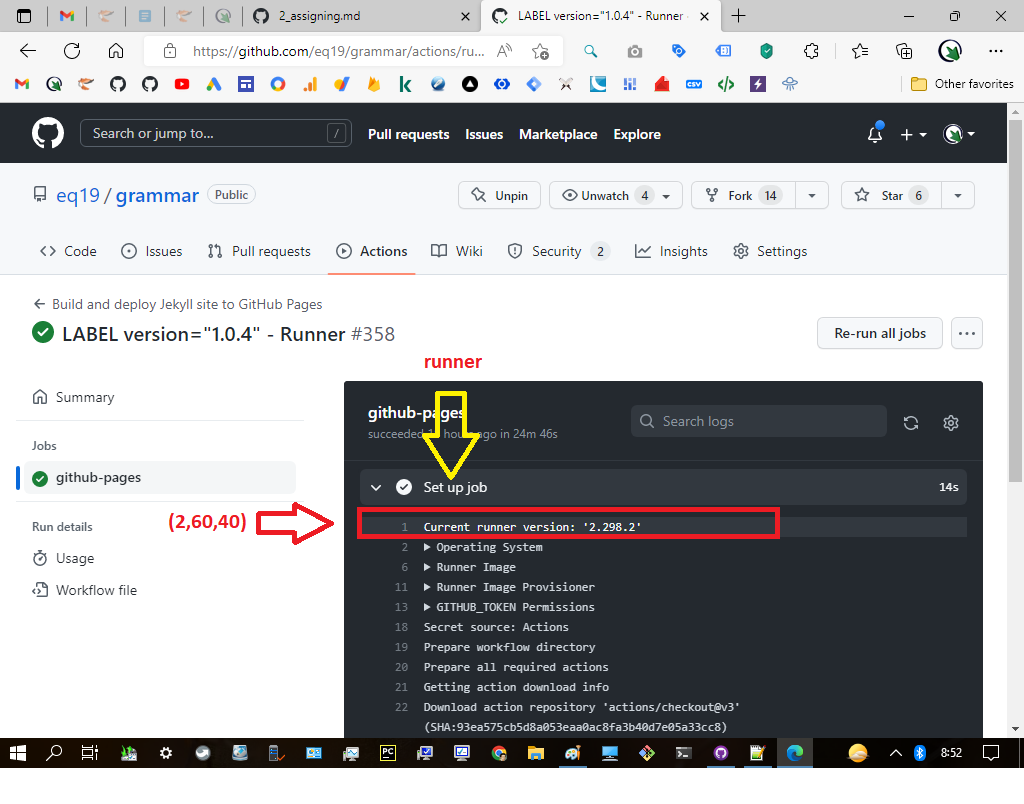By the scheme as we have explained above you would agree that this mapping could only be managed using a customizable compute service so the engine is easily to be created, terminated, restarted and even deleted programmatically.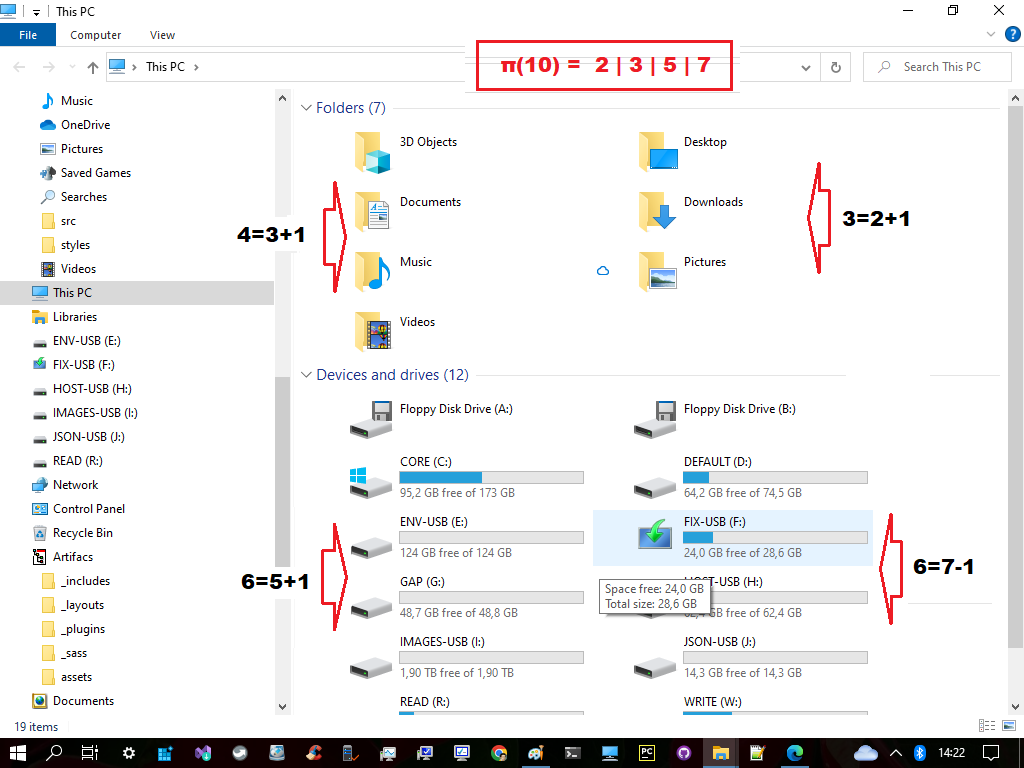See that all of the steps need to be done progressively. Once we buit a container of (2,60,40) with the three (3) inside containers then the 33's will be able to generate four (4) runners that represent (2,3,5,7) from the π(10). Let's discuss them one by one.